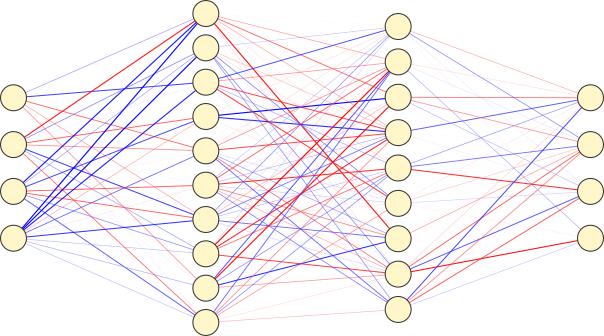## An implementation in PyTorchArtificial neural networks are mainly used for treating data encoded in real values, such as digitized images or sounds. In such systems, using complex-valued tensors would be quite useless. This is however different for physic related topics. When dealing with wave propagation in particular, using complex values is interesting since the physics typically has linear, hence more simple, behavior when considering complex fields. This is sometimes true even when the inputs and the outputs of the system are real values. For instance, consider a complex media that you excite using an amplitude modulator, such as a DMD (Digital Micromirror Device) and you measure the output intensity. You manipulate only real values, but if you want to characterize the system, you have to keep in mind that the phase is a hidden variable as the effect of propagation is represented by the multiplication by a complex matrix on the optical field.

I wrote complexPyTorch a simple implementation of complex-valued functions and modules using the high-level API of PyTorch, allowing building complex-valued artificial neural networks using the guidelines proposed in [C. Trabelsi et al., International Conference on Learning Representations, (2018)]. An up to date version of complexPyTorch, as well as more detailed instructions are available on the complexPyTorch GitHub repository. Please read the documentation there, the following is a simple introduction.

### Context

Deep learning offers new possibilities for physics, especially when dealing with very complex systems, such as disordered systems or non-linear systems (or both!). The ability of artificial neural networks to treat large amounts of degrees of freedom when the underlying model is not totally known is particularly interesting. However, deep learning is not fully model-independent.

While dense layers represent the more general approach, the amount of parameters increases rapidly, limiting their application for large dimension inputs/outputs. It is, in particular, the case for high-resolution images. That is why convolutional layers were introduced. Convolutional networks make the assumption of the locality of the information in images, which can be seen as a priori knowledge about the data. It is a good example of the fact that it is important to introduce the knowledge we have about the data or the system to find an architecture more likely to be efficient with a limited number of parameters to train.

When trying to predict the behavior of a physical system, the closer the architecture of the numerical system is to the physical one, the better.

For wave propagation in linear media, we know that the propagation of light between two planes can be simply represented by a matrix multiplication on the complex optical field. It is then natural to think that complex-valued convolutional or dense layers are a good choice. This approach was used at the University of Glasgow for image transmission through multimode fibers in [O. Moran et al.,  NIPS Proceedings (2018)] and [O. Moral et al, Nat. Commun (2019)]. However, standard frameworks such as TensorFlow or PyTorch do not offer support for complex tensors. For the studies cited, the authors proposed their own code for using Keras and TensorFlow (code here). Being a PyTorch user, I decided to use the flexibility of its high-level API to introduce complex layers.

### Syntax

If you are new to deep learning programming, PyTorch is a very user-friendly framework that copies the Python module numpy in its way to manipulate tensors, which allows a smooth transition if you already use Python for scientific purposes. You would find numerous tutorials online.

The complex modules and functions I introduced copy the standard ones from PyTorch. The names are the same as in nn.modules and nn.functional except that they start with Complex for Modules, e.g. ComplexReluComplexMaxPool2d or complex_ for functions, e.g. complex_relucomplex_max_pool2d. The only usage difference is that the forward function takes two tensors, corresponding to real and imaginary parts, and returns two ones too.

### Example

For illustration, here is a small example of a complex model. Note that in that example, complex values are not particularly useful, it just shows how one can handle complex ANNs.

import torch
import torch.nn as nn
import torch.nn.functional as F
from torchvision import datasets, transforms
from complexLayers import ComplexBatchNorm2d, ComplexConv2d, ComplexLinear
from complexFunctions import complex_relu, complex_max_pool2d

batch_size = 64
trans = transforms.Compose([transforms.ToTensor(),
transforms.Normalize((0.5,), (1.0,))])
train_set = datasets.MNIST('../data', train=True,
test_set = datasets.MNIST('../data', train=False,
shuffle=True)
shuffle=True)

class ComplexNet(nn.Module):     def __init__(self):
super(ComplexNet, self).__init__()
self.conv1 = ComplexConv2d(1, 20, 5, 1)
self.bn  = ComplexBatchNorm2d(20)
self.conv2 = ComplexConv2d(20, 50, 5, 1)
self.fc1 = ComplexLinear(4*4*50, 500)
self.fc2 = ComplexLinear(500, 10)                 def forward(self,x):
xr = x
# imaginary part to zero
xi = torch.zeros(xr.shape, dtype = xr.dtype, device = xr.device)
xr,xi = self.conv1(xr,xi)
xr,xi = complex_relu(xr,xi)
xr,xi = complex_max_pool2d(xr,xi, 2, 2)
xr,xi = self.bn(xr,xi)
xr,xi = self.conv2(xr,xi)
xr,xi = complex_relu(xr,xi)
xr,xi = complex_max_pool2d(xr,xi, 2, 2)

xr = xr.view(-1, 4*4*50)
xi = xi.view(-1, 4*4*50)
xr,xi = self.fc1(xr,xi)
xr,xi = complex_relu(xr,xi)
xr,xi = self.fc2(xr,xi)
x = torch.sqrt(torch.pow(xr,2)+torch.pow(xi,2))
return F.log_softmax(x, dim=1)

device = torch.device("cuda:0" )
model = ComplexNet().to(device)
optimizer = torch.optim.SGD(model.parameters(), lr=0.01, momentum=0.9)

def train(model, device, train_loader, optimizer, epoch):
model.train()
for batch_idx, (data, target) in enumerate(train_loader):
data, target = data.to(device), target.to(device)
output = model(data)
loss = F.nll_loss(output, target)
loss.backward()
optimizer.step()

if batch_idx % 1000 == 0:
print('Train Epoch: {:3} [{:6}/{:6} ({:3.0f}%)]\tLoss: {:.6f}'.format(
epoch,
batch_idx * len(data),
train(model, device, train_loader, optimizer, epoch)